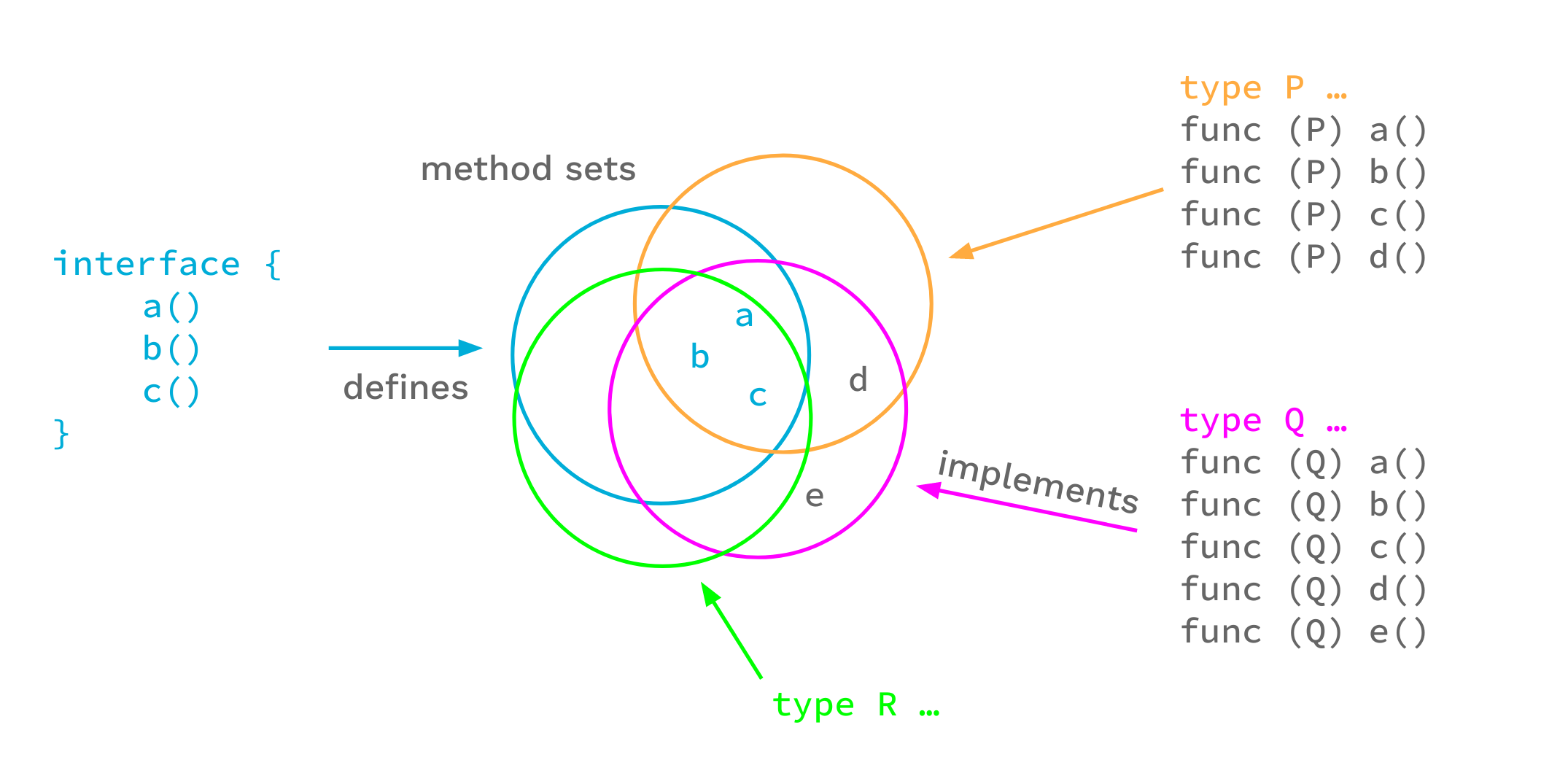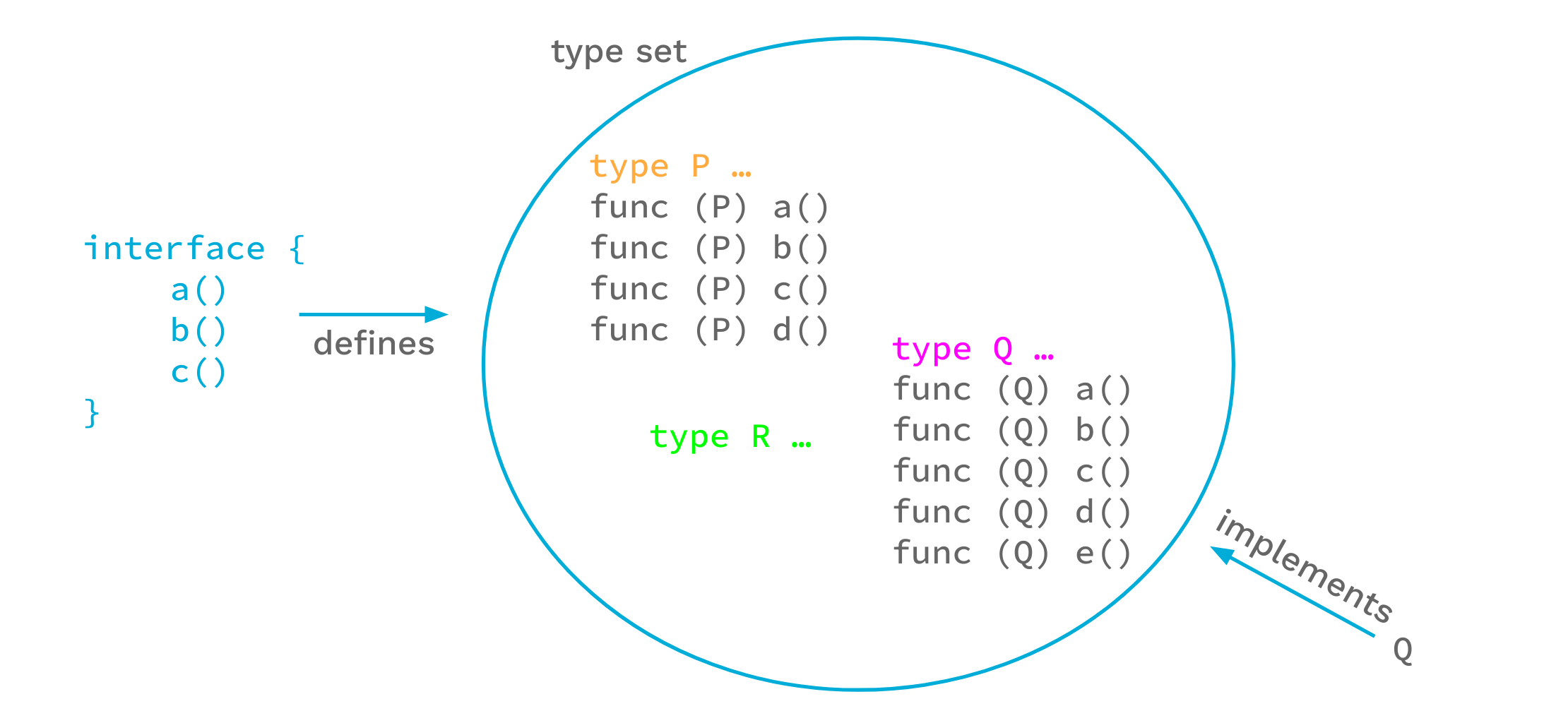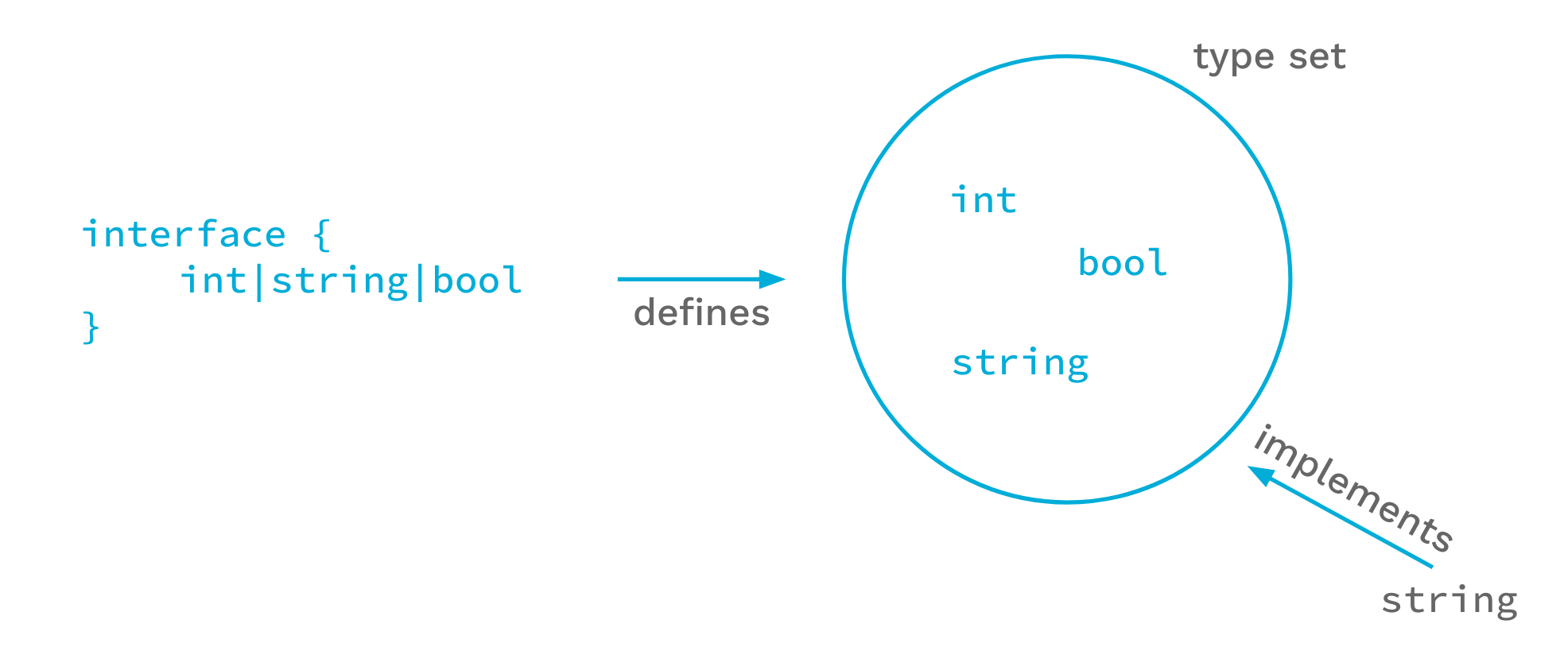## 简介

Go 1.18版本增加了对泛型的支持。泛型是我们自 Go 第一个开源版本以来做出的最大改变。在本文中，我们将介绍新的语言特性。这将不会试图涵盖所有的细节，但我们会点出所有重要的点。更详细的内容，以及许多例子，请参见提案文件。关于语言变化的详细描述，请看更新的语言规范。(请注意，实际上 Go 1.18 的实现对提案文件所允许的内容施加了一些限制，该规范应该是描述准确的。未来的版本可能会取消这部分限制）。

1. 函数和类型的类型参数。
2. 将接口类型定义为类型的集合，包括没有方法的类型。
3. 类型推导，允许在许多情况下调用函数时省略类型参数。

## 类型参数

``````func Min(x, y float64) float64 {
if x < y {
return x
}
return y
}
``````

``````func GMin[T constraints.Ordered](x, y T) T {
if x < y {
return x
}
return y
}
``````

``````x := GMin[int](2, 3)
``````

`GMin` 传入类型参数，在本例中是 `int` ，称为实例化。实例化分为两个步骤。首先，编译器在整个泛型函数或类型中把所有的类型参数替换成它们各自的类型参数。第二，编译器验证每个类型参数是否满足各自的约束条件。我们很快就会知道这意味着什么，但是如果第二步失败，实例化就会失败，程序就会无效。

``````fmin := GMin[float64]
m := fmin(2.71, 3.14)
``````

`GMin[float64]` 的实例化产生了一个有效的原始浮点 `Min` 函数，我们可以在函数调用中使用它。

``````type Tree[T interface{}] struct {
left, right *Tree[T]
value       T
}

func (t *Tree[T]) Lookup(x T) *Tree[T] { ... }

var stringTree Tree[string]
``````

## 类型集合``````type Ordered interface {
Integer|Float|~string
}
``````

``````[S interface{~[]E}, E interface{}]
``````

``````[S ~[]E, E interface{}]
``````

``````[S ~[]E, E any]
``````

## 类型推导

### 函数参数类型推导

``````func GMin[T constraints.Ordered](x, y T) T { ... }
``````

``````var a, b, m float64

m = GMin[float64](a, b) // 显式类型参数
``````

``````var a, b, m float64

m = GMin(a, b) // 没有 类型参数
``````

### 约束类型推导

Go 还支持另一种类型推导，即约束类型推理。为了描述这个，让我们从这个缩放整数切片的例子开始：

``````// Scale 返回s中每个元素都乘以c的副本。
// 这种实现方式有一个问题，后面将会看到。
func Scale[E constraints.Integer](s []E, c E) []E {
r := make([]E, len(s)
for i, v := range s {
r[i] = v * c
}
return r
}
``````

``````type Point []int32

func (p Point) String() string {
// Details not important.
}
``````

``````// ScaleAndPrint 将一个 Point 翻倍，并且打印。
func ScaleAndPrint(p Point) {
r := Scale(p, 2)
fmt.Println(r.String()) // 无法编译
}
``````

``````// Scale 返回s中每个元素都乘以c的副本。
func Scale[S ~[]E, E constraints.Integer](s S, c E) S {
r := make(S, len(s)
for i, v := range s {
r[i] = v * c
}
return r
}
``````